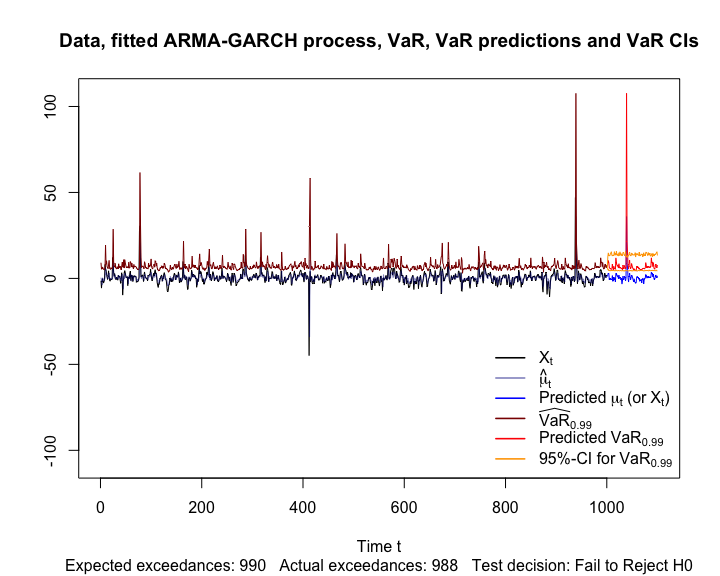# Fitting and Predicting VaR based on an ARMA-GARCH Process

#### 2018-04-28

This vignette does not use qrmtools, but shows how Value-at-Risk (VaR) can be fitted and predicted based on an underlying ARMA-GARCH process (which of course also concerns QRM in the wider sense).

library(qrmtools) # for qq_plot()
library(rugarch)

## 1 Simulate (-log-return) data $$(X_t)$$ from an ARMA-GARCH process

We consider an ARMA(1,1)-GARCH(1,1) process with $$t$$ distributed innovations.

## Setup to simulate from
nu <- 3 # d.o.f. of the standardized distribution of Z_t
fixed.p <- list(mu = 0, ar1 = 0.5, ma1 = 0.3, omega = 4, alpha1 = 0.4, beta1 = 0.2,
shape = nu)
armaOrder <- c(1,1)
garchOrder <- c(1,1)
varModel <- list(model = "sGARCH", garchOrder = garchOrder)
spec <- ugarchspec(varModel, mean.model = list(armaOrder = armaOrder),
fixed.pars = fixed.p, distribution.model = "std")

Simulate one path (for illustration purposes).

## Simulate X_t
n <- 1000 # sample size
## Note: ugarchpath(): simulate from a spec; ugarchsim(): simulate from a fitted object
x <- ugarchpath(spec, n.sim = n, m.sim = 1, rseed = 271) # n = length of simulated path; m = number of paths

## Extract the resulting series
X <- fitted(x) # simulated process X_t = mu_t + epsilon_t for epsilon_t = sigma_t * Z_t
sig <- sigma(x) # volatility sigma_t (conditional standard deviations)
eps <- x@path$residSim # unstandardized residuals epsilon_t = sigma_t * Z_t ## Sanity checks (=> fitted() and sigma() grab out the right quantities) ## Note: There is no such function for epsilon_t stopifnot(all.equal(X, x@path$seriesSim, check.attributes = FALSE),
all.equal(sig, x@path$sigmaSim, check.attributes = FALSE)) As a sanity check, let’s plot the simulated path, conditional standard deviations and residuals. ## Plots plot(X, type = "l", xlab = "t", ylab = expression("Simulated process"~X[t]))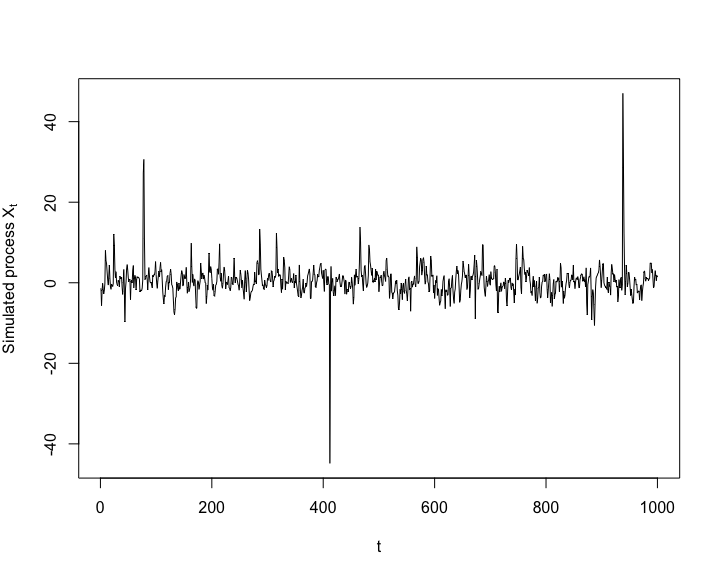plot(sig, type = "l", xlab = "t", ylab = expression("Conditional standard deviation"~sigma[t]))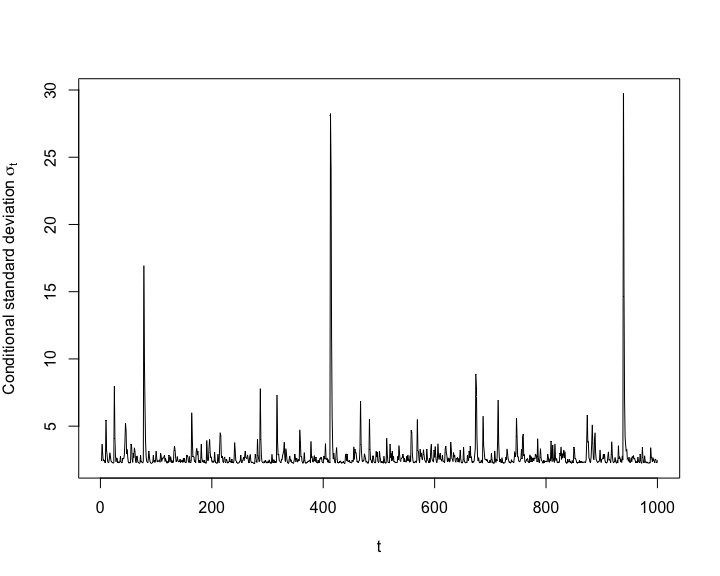plot(eps, type = "l", xlab = "t", ylab = expression("Residuals"~epsilon[t]))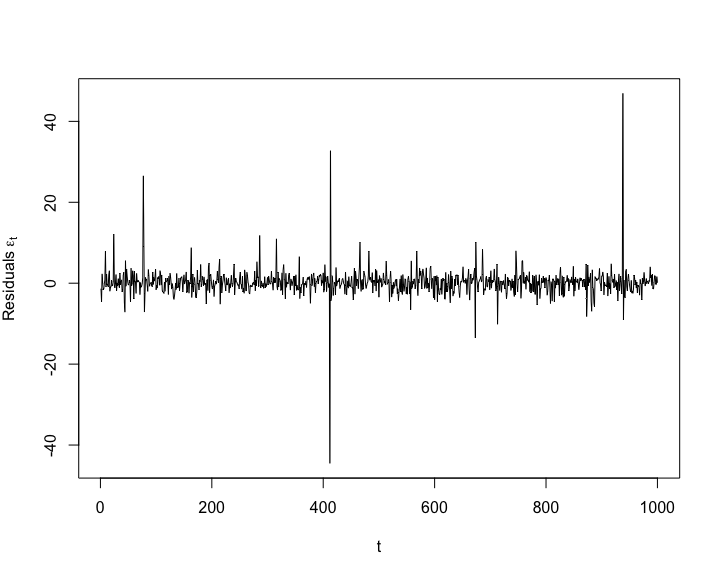## 2 Fit an ARMA-GARCH model to the (simulated) data Fit an ARMA-GARCH process to X (with the correct, known orders here). ## Fit an ARMA(1,1)-GARCH(1,1) model varModel <- list(model = "sGARCH", garchOrder = garchOrder) spec <- ugarchspec(varModel, mean.model = list(armaOrder = armaOrder), distribution.model = "std") fit <- ugarchfit(spec, data = X) # components fit, model ## Extract the resulting series mu <- fitted(fit) # fitted hat{mu}_t (= hat{X}_t) sig <- sigma(fit) # fitted hat{sigma}_t ## Sanity checks (=> fitted() and sigma() grab out the right quantities) stopifnot(all.equal(as.numeric(mu), fit@fit$fitted.values),
all.equal(as.numeric(sig), fit@fit$sigma)) Again let’s consider some sanity checks. ## Data X_t vs fitted hat{mu}_t plot(X, type = "l", xlab = "t", ylab = expression("Data"~X[t]~"and fitted values"~hat(mu)[t])) lines(as.numeric(mu), col = adjustcolor("blue", alpha.f = 0.5)) legend("bottomright", bty = "n", lty = c(1,1), col = c("black", adjustcolor("blue", alpha.f = 0.5)), legend = c(expression(X[t]), expression(hat(mu)[t])))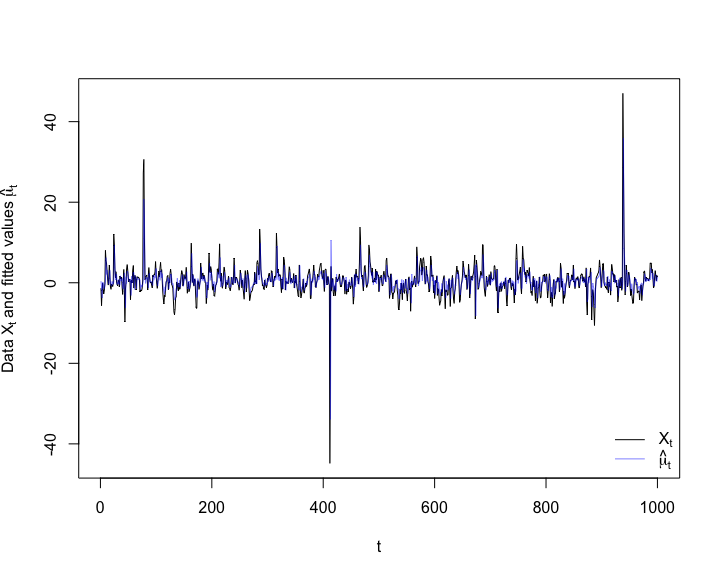## Plot the residuals epsilon_t resi <- as.numeric(residuals(fit)) stopifnot(all.equal(fit@fit$residuals, resi))
plot(resi, type = "l", xlab = "t", ylab = expression(epsilon[t])) # check residuals epsilon_t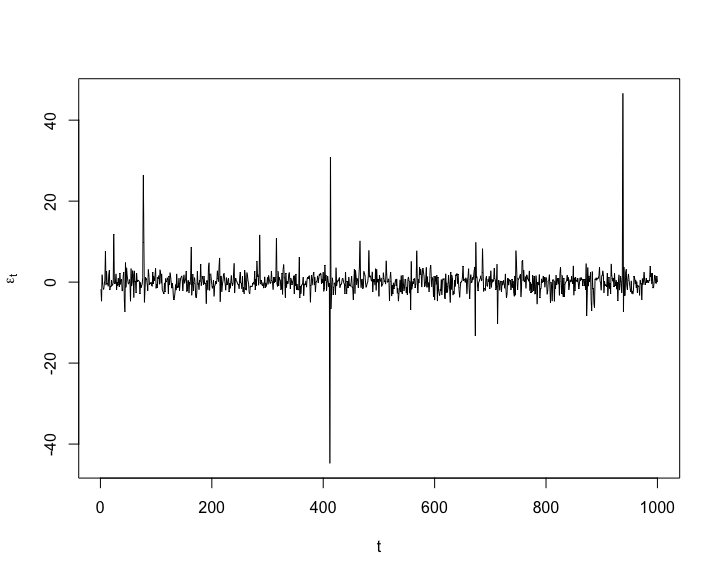## Plot the standardized residuals Z_t
Z <- fit@fit$z stopifnot(all.equal(Z, as.numeric(resi/sig))) qq_plot(Z, FUN = function(p) sqrt((nu-2)/nu) * qt(p, df = nu))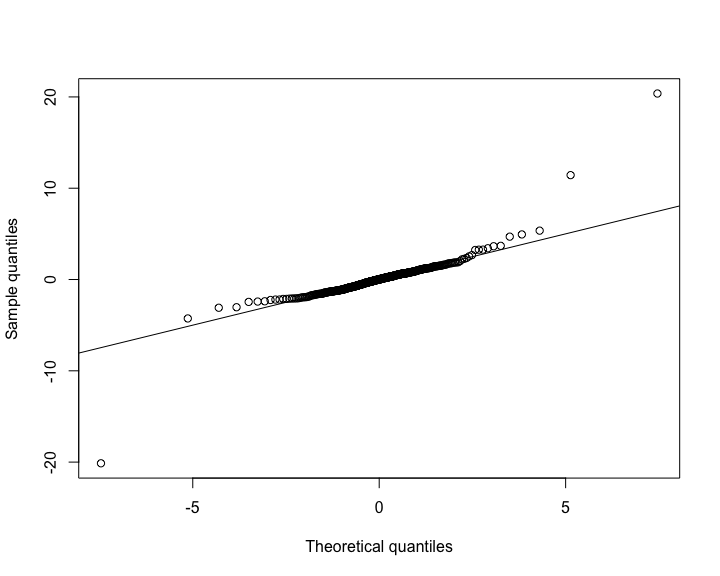## 3 Calculate the VaR time series Compute VaR estimates. Note that we could have also used the GPD-based estimators here. ## VaR estimates and check alpha <- 0.99 # VaR confidence level we consider here VaR <- as.numeric(quantile(fit, probs = alpha)) # a vector (since fit is a rugarch object) nu. <- fit@fit$coef["shape"] # extract (fitted) d.o.f. nu
VaR. <- as.numeric(mu + sig * sqrt((nu.-2)/nu.) * qt(alpha, df = nu.)) # VaR_alpha computed manually
stopifnot(all.equal(VaR., VaR))
## => quantile(<rugarch object>, probs = alpha) provides VaR_alpha = hat{mu}_t + hat{sigma}_t * q_Z(alpha)

## 4 Backtesting via randomness check

Let’s backtest the VaR estimates.

## Backtest VaR_0.99
btest <- VaRTest(alpha, actual = X, VaR = VaR, conf.level = 0.95)
btest$expected.exceed # 0.99 * n ##  990 btest$actual.exceed
##  988
all.equal(sig.predict, pred@forecast$sigmaFor, check.attributes = FALSE)) # sanity check ## Check VaR nu. <- pred@model$fixed.pars$shape # extract (fitted) d.o.f. nu VaR. <- as.numeric(mu.predict + sig.predict * sqrt((nu.-2)/nu.) * qt(alpha, df = nu.)) # VaR_alpha computed manually stopifnot(all.equal(VaR., VaR.predict)) ## 6 Simulate future trajectories of $$(X_t)$$ and compute corresponding VaRs Simulate paths, estimate VaR for each simulated path (note that quantile() can’t be used here so we have to construct VaR manually) and compute bootstrapped confidence intervals for $$\mathrm{VaR}_\alpha$$. ## Simulate B paths B <- 1000 X.boot <- ugarchpath(fspec, n.sim = m, m.sim = B, rseed = 271) # simulate future paths; components path, model, seed ## Bootstrap VaR ## Note: Each series is now an (m, B) matrix (each column is one path of length m) X.t.boot <- fitted(X.boot) # extract simulated X_t sig.t.boot <- sigma(X.boot) # extract sigma_t eps.t.boot <- X.boot@path$residSim # extract epsilon_t
VaR.boot <- (X.t.boot - eps.t.boot) + sig.t.boot * sqrt((nu.-2)/nu.) * qt(alpha, df = nu.) # (m, B) matrix
## => Bootstrapped VaR_alpha computed manually

## Compute bootstrapped two-sided 95%-confidence intervals for VaR
VaR.CI <- apply(VaR.boot, 1, function(x) quantile(x, probs = c(0.025, 0.975)))

## 7 Plot

Finally, let’s display all results.

## Setup
yran <- range(X, mu, VaR, mu.predict, VaR.predict, VaR.CI)
myran <- max(abs(yran))
yran <- c(-myran, myran)
xran <- c(1, length(X) + m)

## Simulated data (X_t) and estimated conditional mean and VaR_alpha
plot(X, type = "l", xlim = xran, ylim = yran, xlab = "Time t", ylab = "",
main = "Data, fitted ARMA-GARCH process, VaR, VaR predictions and VaR CIs",
sub = paste0("Expected exceedances: ", btest$expected.exceed, " Actual exceedances: ", btest$actual.exceed, "   Test decision: ", btest\$uc.Decision))
lines(as.numeric(mu), col = adjustcolor("darkblue", alpha.f = 0.5)) # hat{\mu}_t
lines(VaR, col = "darkred") # estimated VaR_alpha

## Predictions
t. <- length(X) + seq_len(m) # future time points
lines(t., mu.predict, col = "blue") # predicted process X_t (or mu_t)
lines(t., VaR.predict, col = "red") # predicted VaR_alpha
lines(t., VaR.CI[1,], col = "orange") # lower 95%-CI for VaR_alpha
lines(t., VaR.CI[2,], col = "orange") # upper 95%-CI for VaR_alpha
legend("bottomright", bty = "n", lty = rep(1, 6), lwd = 1.6,
col = c("black", adjustcolor("darkblue", alpha.f = 0.5), "blue",
"darkred", "red", "orange"),
legend = c(expression(X[t]), expression(hat(mu)[t]),
expression("Predicted"~mu[t]~"(or"~X[t]*")"),
substitute(widehat(VaR)[a], list(a = alpha)),
substitute("Predicted"~VaR[a], list(a = alpha)),
substitute("95%-CI for"~VaR[a], list(a = alpha))))